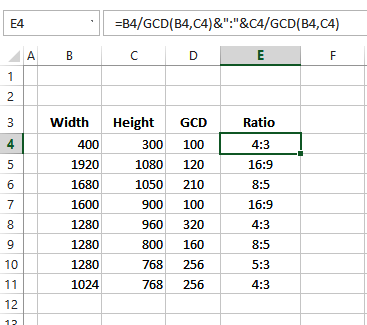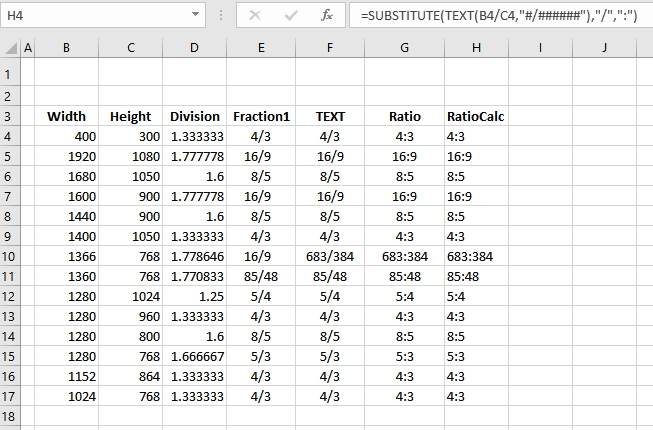## Calculate Ratio with GCD

In this example, there is a list of screen dimensions, with the first width — 400 — in cell B4 and the first height — 300 –in cell C4.

To calculate the ratio for each screen, the formula will use the GCD function, which returns the Greatest Common Divisor, for two or more numbers.

NOTE: In Excel 2003 and earlier, the Analysis Toolpak must be installed, to use the GCD function.

### Test the GCD Function

To see the largest number which will divide equally into the numbers in B4 and C4, enter this formula in cell D4:

=GCD(B4,C4)

The result is 100.

### Create the Ratio Formula

To calculate the ratio, the width will be divided by the GCD and the height will be divided by the GCD. A colon will be placed between those two numbers.

To see the ratio, enter this formula in cell E4:

=B4/GCD(B4,C4)&”:”&C4/GCD(B4,C4)

The result is 4:3 — the ratio for those screen dimensions.## Ratio With TEXT and SUBSTITUTE

Another way to calculate ratio is with the TEXT and SUBSTITUTE functions — these functions work in all versions of Excel, without the Analysis Tookpak having to be installed.

In this example, there is a list of screen dimensions, with the first width — 400 — in cell B4 and the first height — 300 –in cell C4.

To calculate the ratio for each screen, the formula will divide the width by the height, and format the result as a fraction. Then, the slash will be replaced with a colon, to create the ratio.

### Test the TEXT Function

To see the result as a fraction, enter this formula in cell D4:

=TEXT(B4/C4,”#/######”)

The result is 4/3.

### Create the Ratio Formula

To calculate the ratio, the width will be divided by the height, and formatted as a fraction. A colon will replace the slash.

To see the ratio, enter this formula in cell E4:

=SUBSTITUTE(TEXT(B4/C4,”#/######”),”/”,”:”)

The result is 4:3 — the ratio for those screen dimensions.